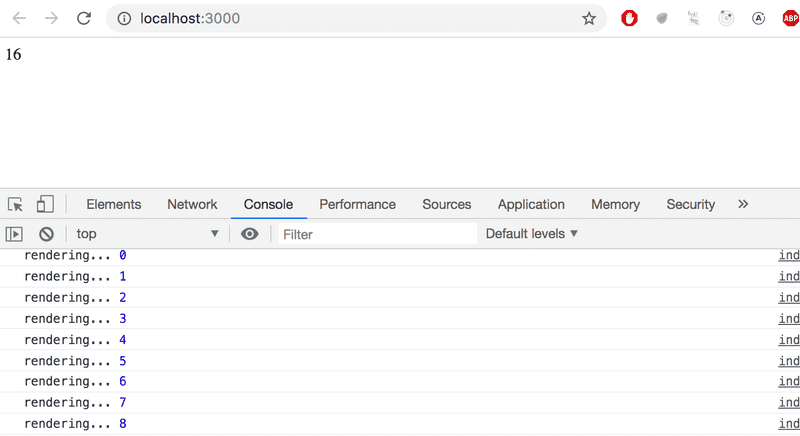c

# 组件状态，事件处理

``````const Hello = (props) => {
return (
<div>
<p>
Hello {props.name}, you are {props.age} years old
</p>
</div>
)
}

const App = () => {
const name = 'Peter'
const age = 10

return (
<div>
<h1>Greetings</h1>
<Hello name="Maya" age={26 + 10} />
<Hello name={name} age={age} />
</div>
)
}``````

### Component helper functions

【组件辅助函数】

``````const Hello = (props) => {
const bornYear = () => {    const yearNow = new Date().getFullYear()    return yearNow - props.age  }
return (
<div>
<p>
Hello {props.name}, you are {props.age} years old
</p>
<p>So you were probably born in {bornYear()}</p>    </div>
)
}``````

### Destructuring

【解构】

``````props = {
name: 'Arto Hellas',
age: 35,
}``````

``````const Hello = (props) => {
const name = props.name  const age = props.age
const bornYear = () => new Date().getFullYear() - age

return (
<div>
<p>Hello {name}, you are {age} years old</p>
<p>So you were probably born in {bornYear()}</p>
</div>
)
}``````

``````const bornYear = () => new Date().getFullYear() - age

const bornYear = () => {
return new Date().getFullYear() - age
}``````

``````const Hello = (props) => {
const { name, age } = props  const bornYear = () => new Date().getFullYear() - age

return (
<div>
<p>Hello {name}, you are {age} years old</p>
<p>So you were probably born in {bornYear()}</p>
</div>
)
}``````

``````props = {
name: 'Arto Hellas',
age: 35,
}``````

``````const Hello = ({ name, age }) => {  const bornYear = () => new Date().getFullYear() - age

return (
<div>
<p>
Hello {name}, you are {age} years old
</p>
<p>So you were probably born in {bornYear()}</p>
</div>
)
}``````

``````const Hello = (props) => {
const { name, age } = props``````

``const Hello = ({ name, age }) => {``

### Page re-rendering

【页面重渲染】

``````import React from 'react'

const App = (props) => {
const {counter} = props
return (
<div>{counter}</div>
)
}

export default App``````

index.js 变成了:

``````import ReactDOM from 'react-dom'
import App from './App'

let counter = 1

ReactDOM.render(
<App counter={counter} />,
document.getElementById('root')
)``````

App 组件通过counter属性，接收到counter的值。 根组件随即将值渲染到屏幕上。 当计数器的值发生变化时会发生什么呢？ 即，如果我们要添加命令

``counter += 1``

``````let counter = 1

const refresh = () => {
ReactDOM.render(<App counter={counter} />,
document.getElementById('root'))
}

refresh()
counter += 1
refresh()
counter += 1
refresh()``````

``````setInterval(() => {
refresh()
counter += 1
}, 1000)``````

### Stateful component

【有状态组件】

``````import ReactDOM from 'react-dom'
import App from './App'

ReactDOM.render(<App />,
document.getElementById('root'))``````

App.js 变成了：

``````import React, { useState } from 'react'
const App = () => {
const [ counter, setCounter ] = useState(0)
setTimeout(    () => setCounter(counter + 1),    1000  )
return (
<div>{counter}</div>
)
}

export default App``````

``import React, { useState } from 'react'``

``const [ counter, setCounter ] = useState(0)``

counter 变量被赋予的初始值state 为零。 变量 setCounter 被分配给一个函数，该函数将用于修改 state

``````setTimeout(
() => setCounter(counter + 1),
1000
)``````

``() => setCounter(counter + 1)``

``````() => {
const [ counter, setCounter ] = useState(0)

setTimeout(
() => setCounter(counter + 1),
1000
)

return (
<div>{counter}</div>
)
}``````

``() => setCounter(2)``

``````const App = () => {
const [ counter, setCounter ] = useState(0)

setTimeout(
() => setCounter(counter + 1),
1000
)

console.log('rendering...', counter)
return (
<div>{counter}</div>
)
}``````### Event handling

【事件处理】

button-元素支持所谓的鼠标事件 ，其中点击是最常见的事件。点击事件同样可能被键盘或者触屏设备所触发，虽然名字叫鼠标事件

``````const App = () => {
const [ counter, setCounter ] = useState(0)

const handleClick = () => {    console.log('clicked')  }
return (
<div>
<div>{counter}</div>
<button onClick={handleClick}>        plus      </button>    </div>
)
}``````

``````const App = () => {
const [ counter, setCounter ] = useState(0)

return (
<div>
<div>{counter}</div>
<button onClick={() => console.log('clicked')}>        plus
</button>
</div>
)
}``````

``````<button onClick={() => setCounter(counter + 1)}>
plus
</button>``````

``````const App = () => {
const [ counter, setCounter ] = useState(0)

return (
<div>
<div>{counter}</div>
<button onClick={() => setCounter(counter + 1)}>
plus
</button>
<button onClick={() => setCounter(0)}>         zero      </button>    </div>
)
}``````

### Event handler is a function

【事件处理是一个函数】

``````<button onClick={() => setCounter(counter + 1)}>
plus
</button>``````

``````<button onClick={setCounter(counter + 1)}>
plus
</button>````````<button onClick={setCounter(counter + 1)}>``

``````<button onClick={() => setCounter(counter + 1)}>
plus
</button>``````

``````const App = () => {
const [ counter, setCounter ] = useState(0)

const increaseByOne = () => setCounter(counter + 1)    const setToZero = () => setCounter(0)
return (
<div>
<div>{counter}</div>
<button onClick={increaseByOne}>        plus
</button>
<button onClick={setToZero}>        zero
</button>
</div>
)
}``````

``````<button onClick={increaseByOne}>
plus
</button>``````

### Passing state to child components

【将状态传递给子组件】

Often, several components need to reflect the same changing data. We recommend lifting the shared state up to their closest common ancestor. 通常，几个组件需要反映相同的变化数据。 我们建议将共享状态提升到它们最接近的共同祖先。

``````const Display = (props) => {
return (
<div>{props.counter}</div>
)
}``````

``````const App = () => {
const [ counter, setCounter ] = useState(0)

const increaseByOne = () => setCounter(counter + 1)
const setToZero = () => setCounter(0)

return (
<div>
<Display counter={counter}/>      <button onClick={increaseByOne}>
plus
</button>
<button onClick={setToZero}>
zero
</button>
</div>
)
}``````

``````const Button = (props) => {
return (
<button onClick={props.onClick}>
{props.text}
</button>
)
}``````

``````const App = () => {
const [ counter, setCounter ] = useState(0)

const increaseByOne = () => setCounter(counter + 1)
const decreaseByOne = () => setCounter(counter - 1)  const setToZero = () => setCounter(0)

return (
<div>
<Display counter={counter}/>
<Button        onClick={increaseByOne}        text='plus'      />      <Button        onClick={setToZero}        text='zero'      />           <Button        onClick={decreaseByOne}        text='minus'      />               </div>
)
}``````

### Changes in state cause rerendering

【状态的改变导致重新渲染】

Display 接收计数器的新值，1，作为props。 Button 组件接收可用于更改计数器状态的事件处理程序，来改变counter的状态。

### Refactoring the components

【重构组件】

``````const Display = (props) => {
return (
<div>{props.counter}</div>
)
}``````

``````const Display = ({ counter }) => {
return (
<div>{counter}</div>
)
}``````

``const Display = ({ counter }) => <div>{counter}</div>``

``````const Button = (props) => {
return (
<button onClick={props.onClick}>
{props.text}
</button>
)
}``````

``````const Button = ({ onClick, text }) => (
<button onClick={onClick}>
{text}
</button>
)``````